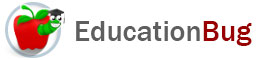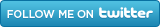PUBLIC SCHOOLS PRIVATE SCHOOLS SCHOOL DISTRICTS COLLEGES PUBLIC LIBRARIES JOBS BLOG RESOURCES
Career Education
Education Choices
Education Costs
Education History and General Information
Education Issues
Education Resources
Family Involvement in Education
Students w Special Needs
Subject Information and Homework Help
Teaching and Learning
Testing
By Types of Schools
By Specific Audiences
General# Math

A collection of articles on some of the fundamental areas of math, including addition, subtraction, multiplication, division, fractions, decimals, rounding numbers, and inequalities, as well as geometric concepts such as area and perimeter.

#### Math Articles

Preparing your child for math can be fun and easy. This article has tips on preparing your child for counting, dividing, comparing, sorting, and measuring. Exposure to all of the these skills can help prepare your child for studying math in school.

Subtraction
Subtraction is one of the main four operations of mathematics you can use to solve a problem, or answer a question. This article has information on forms of subtraction, regrouping or borrowing, and subtracting negative numbers.

Rounding Numbers
Solving math problems may require rounding numbers for a variety of reasons. This article will define a round number, give ways of rounding numbers, and explain rounding numbers by digit.

Number Lines
Number lines are two-dimensional representations of the relationships between numbers. Number lines are like a map of numbers. To understand more about number lines, and what number lines are good for keep reading.

Multiplication
One of the basic math skills everyone needs to know is multiplication. This article defines what multiplication is and how it works, as well as providing information on multiplication tables and how to use them. Keep reading for more on multiplication.

Decimals
Decimals are numbers written using the decimal system. The decimal system is based on powers of 10 and assigned places, so that the position of a digit with respect to the decimal point is indicative of its value. For more about decimals, keep reading.

Measurement
Three of the most common types of measurement that people do are measurement of distance, area, and time, so those are the three discussed in this article.. Keep reading for more on systems of measurement and conversions.

Division
Division is the inverse of multiplication. This article has information on several different types of division including horizontal division and long division. Also, tips on special division, remainders, decimals, and repeating decimals.

By the time weâ€™re adept at it, addition may seem like old hat. But actually, there are different ways to do addition, and we might choose different approaches, depending on our purposes. Keep reading for explanations of different ways to do addition.

Math Science Projects
Math science projects are often overlooked when considering science fair projects. This article has information, tips, and ideas for fun and easy math science projects. Consider making your next science fair project a math science project.

Computer Science Projects
These computer science projects can be modified or adapted to suit the purposes of a homeschool class, a public or private school project, or a science fair project. Keep reading for more on computer science project ideas and tips.

Math Symbols
That math problems in words are labeled â€œword problemsâ€ is  reminder to us that it is typical for mathematical communication to be made without words, so it's critical to understand  the meanings of math symbols. Read on for more information about common math symbols.

Fractions
This article takes a look at fraction basics: what a fraction is, different ways to represent fractions, and how to work with fractions, turning them into decimals and percentages, for example, and converting fractions by finding the Least Common Denominator (LCD).

Kindergarten Math
If youâ€™re looking for ways to support your public or private school kindergartner in learning math, or formulating your own homeschool math curriculum, youâ€™ll find useful information in this article. Read on to learn about all the different elements of kindergarten math.

Perimeter
In this article about perimeter, what perimeter is and how to calculate perimeter are both explained. Learn how to find the perimeter of a polygon, whether equilateral or not, and how to find both the perimeter, or circumference, and area of a circle.

Area
Read this article to learn more about what area is, how to find area, and how to express the results once you have found the area. Get some basic formulas for finding area of triangles, circles, rectangles, squares, and parallelograms.

Homeschool Math
Maybe math was your favorite subject in school and maybe it wasnâ€™t. However you feel about math, itâ€™s important to have some familiarity in order to teach it to your child. Hereâ€™s an article to help you think about how to get started with homeschool math.

Inequalities
What is an inequality, what different types of inequalities exist, and how can you use them? Learn the answers to these questions about inequalities and more in this article.

Roman Numerals
Roman numerals are symbols that represent numbers. Read this article for more information on how Roman numerals are organized and used. Also, see a chart showing the Roman numerals from one to one million.